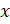### CO-ORDINATE GEOMETERY 1

Mathematics SSS 3 First Term

WEEK 9

CO-ORDINATE GEOMETERY 1

Performance Objectives

Student should be able to:

1. Identify Cartesian rectangular coordinate (x, y).
2. Draw and interpret linear graph (Straight line graph).
3. Calculate the distance between two pints.
4. Calculate the midpoint of a line joining two points.

Content

Cartesian Rectangular Coordinate

Where trying to draw a graph, it is important to have two fixed reference line called coordinate axis. One is horizontal and the other is vertical, and they intersect one another at right angles. The horizontal line is generally known as thecoordinate or the x View More